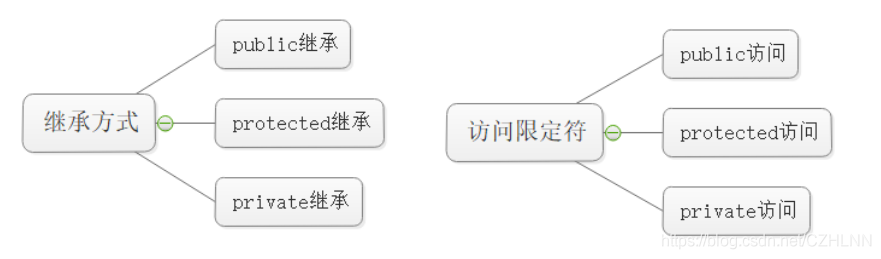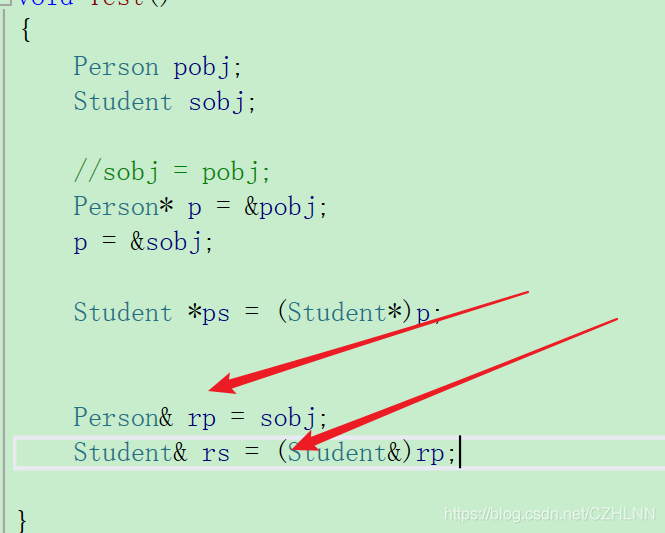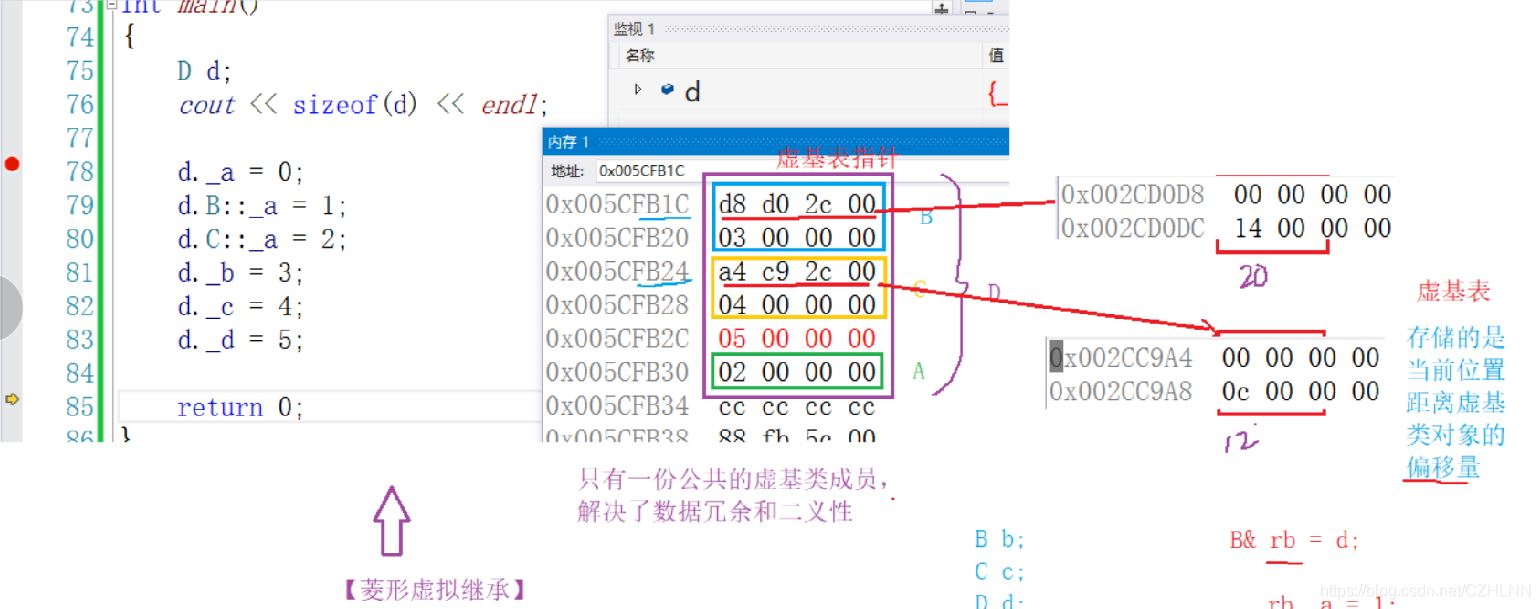﻿ 老生常谈C++ 中的继承_C 语言_脚本之家

# 老生常谈C++ 中的继承

更新时间：2021年04月30日 10:38:09   作者：一枚快乐的野指针

## 1 什么是继承

### 1.1 继承的概念

```#include <iostream>
#include <string>

using namespace std;

class Person
{
public:
void Print()
{
cout << "name:" << _name << endl;
cout << "age:" << _age << endl;
}
protected:
string _name = "peter"; // 姓名
int _age = 18;
};
class Student : public Person
{
protected:
int _stuid;
};
int main()
{
Person p;
Student s;
p.Print();
s.Print();
return 0;
}```## 1.2 继承的定义

### 1.2.1 定义格式

Person是父类，也称作基类。Student是子类，也称作派生类。1.2.2 继承关系和访问限定符1.2.3 继承基类成员访问方式的变化• 基类 private 成员在派生类中无论以什么方式继承都是不可见的。这里的不可见是指基类的私有成员还是被继承到了派生类对象中，但是语法上限制派生类对象不管在类里面还是类外面都不能去访问它。
• 基类 private 成员在派生类中是不能被访问，如果基类成员不想在类外直接被访问，但需要在派生类中能访问，就定义为protected。可以看出保护成员限定符是因继承才出现的。
• 实际上面的表格我们进行一下总结会发现：基类的私有成员在子类都是不可见的。基类的其他成员在子类的访问方式 = Min(成员在基类的访问限定符，继承方式)，public > protected > private。
• 使用关键字class时默认的继承方式是private，使用struct时默认的继承方式是public，不过最好显示的写出继承方式。
• 在实际运用中一般使用都是public继承，几乎很少使用protetced/private继承，也不提倡使用protetced/private继承，因为protetced/private 继承下来的成员都只能在派生类的类里面使用，实际中扩展维护性不强。

## 2 基类(父类)对象和派生类(子类)对象之间的赋值转换

•  is a 的关系：一个子类是一个父类，比如，一个学生是一个人
• 派生类对象 可以赋值给 基类的对象 / 基类的指针 / 基类的引用。这里有个形象的说法叫切片或者切割。寓意把派生类中父类那部分切来赋值过去（重点掌握），代码演示如下。
```#include <iostream>
#include <string>
using namespace std;

class Person
{
public:
// 缺省
string _name = "陈志浩"; // 姓名
string _sex = "男"; // 性别
int _age = 25; // 年龄
};
class Student : public Person
{
public:
int _No = 2019124084; // 学号
};

void Test()
{
Person pobj;
Student sobj;
// 修改子类的成员变量方便演示
sobj._name = "chenzhiiao";
sobj._age = 23;
}
int main()
{
Test();
return 0;
}```## 3 继承中的作用域

• 在继承体系中基类和派生类都有独立的作用域
• 子类和父类中有同名成员，子类成员将屏蔽对父类的同名成员的直接访问，这种情况叫隐藏，也叫重定义。注意不叫重载也不叫重写，重载要在同一个作用域才可以。（在子类成员函数中，可以使用 基类::基类成员 显示访问）
• 需要注意的是如果是成员函数的隐藏，只需要函数名相同就构成隐藏，即使形参列表和函数体不同也是构成隐藏的哦。
• 注意在实际中在继承体系里面最好不要定义同名的成员。

```#include <iostream>
using namespace std;

class A {
public:
void fun()
{
cout << "func()" << endl;
}
};
class B : public A {
public:
void fun(int i)
{
cout << "func(int i)->" << i << endl;
}
};
void Test()
{
// B中的fun和A中的fun不是构成重载，因为不是在同一作用域
// B中的fun和A中的fun构成隐藏，成员函数满足函数名相同就构成隐藏。
B b;
b.fun(10);
b.A::fun();//可以使用 基类::基类成员 显示访问
};
int main()
{
Test();
return 0;
}```## 4 派生类(子类)的默认成员函数

```#include <iostream>
#include <string>
#include <ostream>
using namespace std;
class Person
{
public:
friend ostream& operator<<(ostream& out, const Person& p);
Person(const char* name = "peter")
: _name(name)
{
cout << "Person()" << endl;
}

Person(const Person& p)
: _name(p._name)
{
cout << "Person(const Person& p)" << endl;
}

Person& operator=(const Person& p)
{
cout << "Person& operator=(const Person& p)" << endl;
if (this != &p)
_name = p._name;

return *this;
}

~Person()
{
cout << "~Person()" << endl;
}
protected:
string _name; // 姓名
};
ostream& operator<<(ostream& out, const Person& p)
{
out << "name : " << p._name << endl;
return out;
}
class Student : public Person{
public:
friend ostream& operator<<(ostream& out, const Student& s);
Student(const char* name = "chenzhiao", int no = 2019)
:Person(name)
,_no(no)
{
cout << "Student()" << endl;
}

~Student()
{
cout << "~Student()" << endl;
}

protected:
int _no;
};
ostream& operator<<(ostream& out, const Student& s)
{
out << "name : " << s._name << ",no : " << s._no << endl;
return out;
}

void Test()
{
Student s1;
cout << s1;
};
int main()
{
Test();
return 0;
}``````Student(const Student& s)
: Person(s), _no(s._no)
{
cout << "Student(const Student& s)" << endl;
}``````Student& operator=(const Student& s)
{
if (this != &s)
{
Person::operator=(s);
//这里必须要用类名指定，否则子类的operator=会把父类的
//的operator=隐藏掉，导致无限递归调用子类的operator=导致栈溢出
_no = s._no;
}
return *this;
}```

```~Student()
{
cout << "~Student()" << endl;
Person::~Person();
//C++的编译器保证了这个代码默认会执行，但是必须要放在最后一行才符合规则
//不能让程序员去手动指定Person::~Person();必须要放在最后一行
//万一程序员不小心放在了第一行，会导致一些不可预料的错误，所以不要显示调用
//这和上面3个默认成员的规则不一样，需要多注意
}```## 6 继承与静态成员

```#include <iostream>
#include <string>
using namespace std;

class Person
{
public:
Person() { ++_count; }
protected:
string _name; // 姓名
public:
static int _count; // 统计人的个数。
};
int Person::_count = 0;
class Student : public Person
{
protected:
int _stuNum; // 学号
};
{
protected:
string _seminarCourse; // 研究科目
};
void TestPerson()
{
Student s1;
Student s2;
Student s3;
Person s5;
cout << " 人数 :" << Person::_count << endl;
Student::_count = 0;
cout << " 人数 :" << Person::_count << endl;
}
int main()
{
TestPerson();
return 0;
}```

## 7 复杂的菱形继承以及菱形虚拟继承

### 7.1 单继承### 7.2 多继承### 7.3 菱形继承### 7.3.1 菱形继承的问题

```#include <iostream>
#include <string>
using namespace std;

class Person{
public:
string _name;
};
class Student : public Person{
protected:
int _stuId;
};
class Teacher : public Person{
protected:
int _teaId;
};
class Assistant : public Student, public Teacher{
protected:
string _majorCourse;
};
void Test()
{

Assistant a;
//a._name = "chenzhiao"; 这样会有二义性无法明确知道访问的是哪一个

// 需要显式指定访问哪个父类的成员可以解决二义性的问题，但是数据冗余的问题还是无法解决

a.Student::_name = "xxx";
a.Teacher::_name = "yyy";

}
int main()
{
Test();
return 0;
}```### 7.3.2 如何解决

```#include <iostream>
#include <string>
using namespace std;

class Person{
public:
string _name;
};
class Student : virtual public Person{
protected:
int _stuId;
};
class Teacher : virtual public Person{
protected:
int _teaId;
};
class Assistant : public Student, public Teacher{
protected:
string _majorCourse;
};
void Test()
{

Assistant a;
a._name = "chenzhiao";

}
int main()
{
Test();
return 0;
}
// 在菱形继承的“肩膀处”添加完 virtual 关键字，完成虚拟继承，可以解决数据的二义性和冗余问题。我们来看一下监视窗口```### 7.3.3 虚拟继承解决数据冗余和二义性的原理

为了研究虚拟继承原理，我们给出了一个简化的菱形继承体系，再借助内存窗口观察对象成员的模型。```#include <iostream>
#include <string>
using namespace std;

class A {
public:
int _a;
};
// class B : public A
class B : public A {
public:
int _b;
};
// class C : public A
class C : public A {
public:
int _c;
};
class D : public B, public C {
public:
int _d;
};

void Test()
{
D d;
cout << sizeof(d) << "字节" << endl;
d.B::_a = 1;
d.C::_a = 2;
d._b = 3;
d._c = 4;
d._d = 5;

}
int main()
{
Test();
return 0;
}```• 可以分析出 D对象中将 A 放在了对象组成的最下面，这个 A 同时属于 B 和 C，那么 B 和 C 如何找到公共的 A 呢？这里是通过了 B 和 C 的虚基表指针，这两个指针指向了虚基表，**虚基表存的是当前位置距离 虚基类对象（A）的偏移量，通过偏移量找到A。尤其是发生切片行为的时候，比如 B& b = d，这个偏移量会帮助 b 找到自己的a，完成切片行为。注意：菱形虚拟继承的效率并不高，因为这一顿操作可想而知，所以尽量不要定义菱形虚拟继承，这也是 C++ 语法设计的几个大 bug。
• **下面是上面的Person关系菱形虚拟继承的原理解释：## 8 继承的扩展和总结

• 多继承就是C++语法复杂的体现，有了多继承就有了菱形继承，有了菱形继承就有了菱形虚拟继承，底层实现就很复杂，所以不建议设计多进程，更不要设计出菱形继承。否则在复杂度及性能上都有问题。
• 可以认为多继承就是 C++ 的缺陷之一，很多后来的OO语言都没有多继承，如Java。

### 8.1 继承和组合

• public继承是一种is-a的关系。也就是说每个派生类对象都是一个基类对象，比如 狗是一种动物
• 组合是一种has-a的关系。假设B组合了A，每个B对象中都有一个A对象。
• 继承允许你根据基类的实现来定义派生类的实现。这种通过生成派生类的复用通常被称为白箱复用，(white-box reuse)。术语“白箱”是相对可视性而言：在继承方式中，基类的内部细节对子类可见 。继承一定程度破坏了基类的封装，基类的改变，对派生类有很大的影响。派生类和基类间的依赖关系很强，耦合度高。
• 对象组合是类继承之外的另一种复用选择。新的更复杂的功能可以通过组装或组合对象来获得。对象组合要求被组合的对象具有良好定义的接口。这种复用风格被称为黑箱复用(black-box reuse)，因为对象的内部细节是不可见的。对象只以“黑箱”的形式出现。 组合类之间没有很强的依赖关系，耦合度低。优先使用对象组合有助于你保持每个类被封装。
• 实际尽量多去用组合。组合的耦合度低，代码维护性好。不过继承也有用武之地的，有些关系就适合继承那就用继承，另外要实现多态，也必须要继承。类之间的关系可以用继承，可以用组合，就用组合。